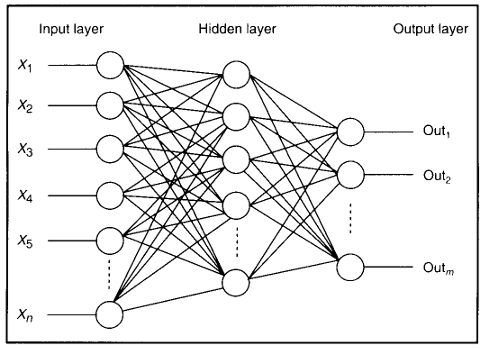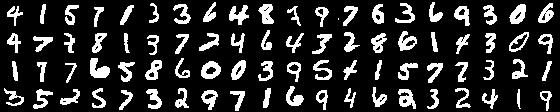## Deep Learning in R using H2O

#### By Devin Didericksen on October 17, 2014### Introduction

While there are a few good options in python for deep learning neural networks, I wanted to find a solution in R that would support the following six features:

1. Deep architectures of several hidden layers
2. Parallelization across GPU cores
3. Dropout
4. Activation function options that include Sigmoid, Rectified Linear Units (ReLU), Hyperbolic Tangent, and Maxout
6. Convolutions and Max Pooling

Through searching, I discovered that there are essentially only three R packages for deep learning: darch, deepnet, and h2o. None of the three satisfy the last requirement (so no convolutional neural networks in R at the moment), and only h2o satisfies the other five requirements.

### H2O

As I looked into H2O I found a lot of things I really liked:

• It is a scalable in-memory platform for machine learning.
• It is free and open source.
• It is cross-platform and supports R, Python, Scala, and Java.
• Last but not least, it has a dedicated team of developers continually making improvements to their product.

I decided to brush up on H2O by building a deep learning model using some of the latest advancements such as dropout and ReLU.

### Classification of Handwritten Digits

The dataset I’ll be using to train my deep neural network is MNIST – the most famous computer vision dataset consisting of pixel intensities of 28 X 28 images of handwritten digits. I decided to use Kaggle’s training and test sets of MNIST. The training set has 42,000 records with 784 inputs (each pixel in a 28 X 28 image) and a label for each record indicating the digit. The test set has 28,000 records. This is a 60/40 split vs. the 86/14 split used in the benchmarks tracked by Yann Lecun, so we will have to keep this in mind when comparing results. 18,000 less records in the training set will definitely drag down the classification accuracy.#### H2O Initialization and Data Import

The first step is to start an instance of H2O. Using the “Xmx” parameter in the h2o.init function we can set aside the amount of RAM we want to use. I have 4 GB of RAM on my machine so I allocated 3 GB to H2O. Since the pixel intensity ranges from 0 and 255 I can easily scale my data by dividing all inputs by 255.

library(h2o)
localH2O = h2o.init(ip = "localhost", port = 54321, startH2O = TRUE, Xmx = '3g')
train <- h2o.importFile(localH2O, path = "data/train.csv")
train <- cbind(train[,1],train[,-1]/255.0)
test <- h2o.importFile(localH2O, path = "data/test.csv")
test <- test/255.0

#### Building the Model

When defining parameters for the deep neural network I used many of the suggestions in Geoffrey Hinton’s and Alex Krizhevsky’s paper. The model I settled on has the following attributes:

• Rectified Linear Units as the activation function
• Input dropout ratio of 0.2
• Hidden dropout ratio of 0.5
• Neuron architecture of 784-800-800-10 (784 inputs, 2 hidden layers of 800 neurons each, and 10 softmax output neurons)
• 500 epochs
s <- proc.time()
set.seed(1105)
model <- h2o.deeplearning(x = 2:785,
y = 1,
data = train,
activation = "RectifierWithDropout",
input_dropout_ratio = 0.2,
hidden_dropout_ratios = c(0.5,0.5),
balance_classes = TRUE,
hidden = c(800,800),
epochs = 500)
e <- proc.time()
d <- e - s
d
model

The section of code just above took 29.5 hours to run on my machine (which has a low-end single core GPU). I’m sure if I had a decent multi-core GPU the running time would have been greatly reduced. Once the model was done training I inspected the confusion matrix. Interestingly enough, almost all the prediction errors (97.2%) on the training set are because the model predicted an “8” when the digit was something else.

#### Making Predictions on the Test Set

After training the model we can pass the test set through the model to create a prediction array. This array was written out in a csv in a format acceptable for a Kaggle submission.

yhat <- h2o.predict(model, test)
ImageId <- as.numeric(seq(1,28000))
names(ImageId) <- "ImageId"
predictions <- cbind(as.data.frame(ImageId),as.data.frame(yhat[,1]))
names(predictions) <- "Label"
write.table(as.matrix(predictions), file="DNN_pred.csv", row.names=FALSE, sep=",")

### Kaggle Submission Result & Conclusion

When I submitted the csv to Kaggle, I received a result of 96.2% on the leaderboard (which is calculated on 25% of the test data). Initially I was a little disappointed in this result, until I realized the models benchmarked on Yann Lecun’s site were trained with almost 50% more data. If I were to spend more time to increase the accuracy of the model, I would try ensembling, increasing the size of the data set by a factor of five through shifting each image by one pixel in all four directions, adding convolution, and tuning the neuron architecture.

Going through this exercise of building a deep learning model has been a good experience, and it has inspired me to invest in a GPU. I look forward to building deep learning models in the future for other use cases. I was also impressed with H2O. It appears to be a promising machine learning platform, and I plan to explore its other features and machine learning models.